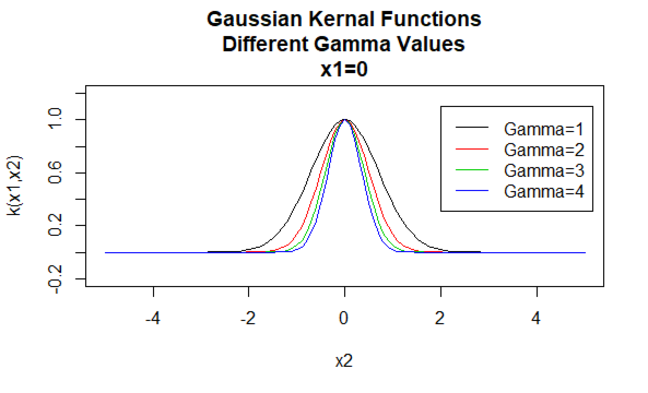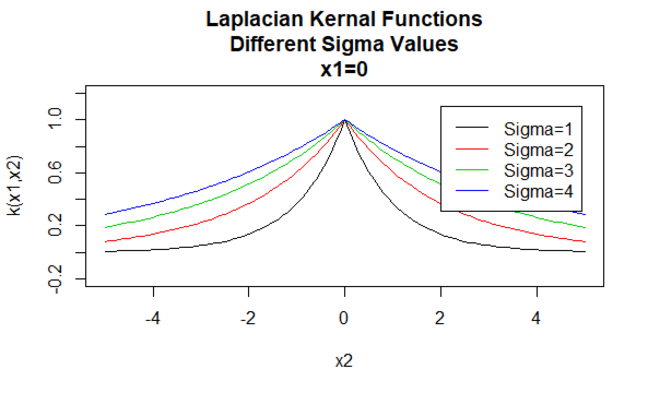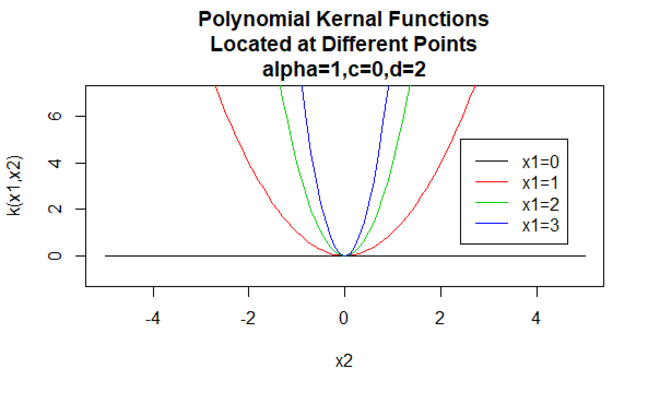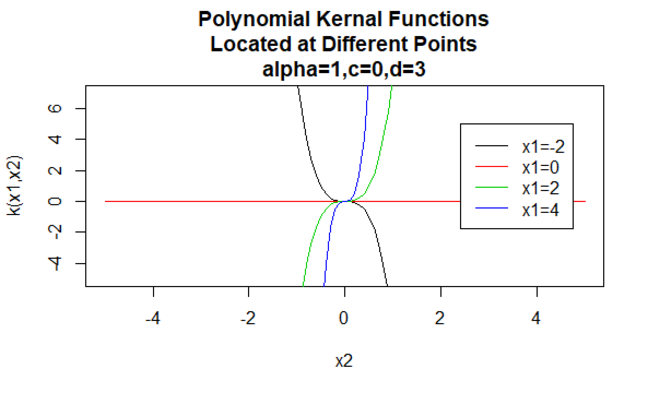## Want to keep learning?

This content is taken from the The Open University & Persontyle's online course, Advanced Machine Learning. Join the course to learn more.
3.2

# Basics & Kernel Regression

We begin by looking at dual-form linear/ridge regression, before showing how to ‘kernelize’ it. In explaining the latter, we will see what kernels are, and what the ‘kernel trick’ is.

## Dual-form Ridge Regression

Linear regression is typically given in it primary form as a linear combination of columns (features). However, there exists a second, dual form where it is a linear combination of the inner product of a new datum (which we are performing inference on) with each of the training data.

We consider the case of ridge regression (L2 regularized linear regression), remembering that basic linear regression corresponds to the case where $$\lambda = 0$$. Then the formulas for ridge regression, where $$X$$ and $$Y$$ refer to the $$n \times m$$ training data and $$x^\prime,y^\prime$$ a new case to be estimated, are:

$\hat y^\prime = \sum_{i=0}^{m} \beta_i x^\prime_i$ $\beta = ( X^TX + \lambda I )^{-1} X^TY$ $\hat y^\prime = \sum_{i=0}^{n} \alpha_i \langle X_i,x^\prime \rangle$ $\alpha = ( XX^T + \lambda I )^{-1} Y$

Where $$\langle X_i,x^\prime \rangle$$ is the inner/dot product, so $$\langle X_i,x^\prime \rangle = X^T_i x^\prime = \sum_j^m X_{i,j} x^\prime_j$$.

The dual form shows that linear/ridge regression can also be understood as providing an estimate of a weighted sum of the inner product of a new case with each of the training cases.

It means we can do linear regression even when there are more columns than rows, though the importance of this can be overstated since (i) we can do this anyway via the use of L2 regularization since this always makes the $$X^TX$$ matrix invertible; and (ii) the $$XX^T$$ matrix can often require L2 regularization anyway to ensure numerical stability for the inversion. It also allows us to view linear regression as much more of a sequential learning process, where each additional datum in the training data brings something new.

Most importantly for our purposes, though, the dual form has the interesting characteristic: feature vectors occur in the equations only inside inner products. This is true even in the definition of $$\alpha$$, as $$XX^T$$ produces the matrix corresponding to the inner products of every pair of feature vectors in the training data. We will see the importance of this as we proceed.

Aside: Interested students can see how the dual form was derived in the Derivation of Dual Form document available in the downloads section at the end of this article.

### Non-Linear Dual Ridge Regression

We can turn our dual form ridge regression into a non-linear model by the standard method of using a non-linear feature transformations $$\phi$$:

$\hat y^\prime = \sum_{i=0}^{n} \alpha_i \langle \phi(X_i),\phi(x^\prime) \rangle$ $\alpha = ( \phi(X)\phi(X)^T + \lambda I )^{-1} Y$

### Kernel Functions

A kernel function, $$K: \mathcal X \times \mathcal X \to \mathbb{R}$$, is a function that is symmetric - $$K(x_1,x_2)=K(x_2,x_1)$$ - and positive definite (see the aside for a formal definition). Positive-definiteness is used in the mathematics that justifies the use of kernels. But without significant mathematical knowledge, the definition is not intuitively illuminating. So rather than attempting to understand kernels from the definition of positive-definiteness, we will introduce them with a number of examples.

Before doing this, we note that although kernels are two-argument functions, it is common to think of them as being located at their first argument, and being a function of their second. According with this interpretation you will see notation such as $$K_x(y)$$, which is equivalent to $$K(x,y)$$ . In particular, we will often think of kernels being single argument functions ‘located’ at data points (feature vectors) in our training data. Sometimes you will read of us ‘dropping’ kernels on data points. So if we have a feature vector $$x_i$$ , we would drop a kernel on it, leading to the function $$K_{x_i}(x)$$ located at $$x_i$$ and equivalent to $$K(x_i,x)$$ .

We also note that kernels are often specified as members of parametric families. Examples of such kernel families include:

#### Gaussian Kernels

Gaussian kernels are an example of radial basis function kernels and are sometimes called radial basis kernels. The value of a radial basis function kernel depends only on the distance between the argument vectors, rather than their location. Such kernels are also termed stationary.

Parameters: $$\gamma$$

Equation form: $$K(X_1,X_2)=e^{-\gamma \| X_1 - X_2 \|^2}$$

#### Laplacian Kernels

Laplacian kernels are also radial basis functions.

Parameters: $$\sigma$$

Equation form: $$K(X_1,X_2)=e^{-\frac{\| X_1 - X_2 \|}{\sigma}}$$

#### Polynomial Kernels

Polynomial kernels are an example of non-stationary kernels. So these kernels will assign different values to pairs of points that share the same distance, based on their values. Parameter values must be non-negative to ensure these kernels are positive definite.

Parameters: $$\alpha , c , d$$

Equation form: $$K(X_1,X_2)=(\alpha X_1^TX_2 +c)^d$$

Specifying particular values for the parameters of a kernel family results in a kernel function. Below are examples of kernels functions from the above families with particular parameter values located at different points (i.e. the plotted graph is a function of the second argument, with the first argument set to a specific value).Aside: Interested students can see the definition of positive definiteness for kernels in the Kernels and Positive Definiteness document available in the downloads section at the end of this article.

### The Kernel Trick

The importance of kernel functions comes from a very special property: Every positive-definite kernel, $$K$$ is related to a mathematical space, $$\mathcal{H}_K$$, (known as the reproducing kernel Hilbert space (RKHS) of the kernel) such that applying $$K$$ to two feature vectors, $$X_1,X_2$$ is equivalent to projecting these feature vectors into $$\mathcal{H}_K$$ by some projection function, $$\phi$$ and taking their inner product there:

$K(X_1,X_2)=\langle \phi(X_1),\phi(X_2) \rangle_{H_K}$

The RKHSs associated with kernels are typically high-dimensional. For some kernels, like Gaussian family kernels, they are infinite dimensional.

The above is the basis for the famous ‘kernel trick’: If the input features are involved in the equation of a statistical model only in the form of inner-products then we can replace the inner-products in the equation with calls to the kernel function and the result is as if we had projected the input features into a higher dimensional space (i.e. performed a feature transformation leading to a large number of latent variable features) and taken their inner-product there. But we never need to perform the actual projection.

In machine learning terminology, the RKHS associated with the kernel is known as the feature space, as opposed to the input space. Via the kernel trick we implicitly project the input features into this feature space and take their inner product there.

### Kernel Regression

This leads to the technique known as kernel regression. It is simply an application of the kernel trick to the dual form of ridge regression. For ease we introduct the idea of the Kernel, or Gram, matrix, $$K$$, such that $$K_{i,j}=k(X_i,X_j)$$. Then we can write the equations for kernel regression as:

$\hat y^\prime = \sum_{i=0}^{n} \alpha_i k(X_i,x^\prime)$ $\alpha = ( K + \lambda I )^{-1} Y$

Where $$k$$ is some positive-definite kernel function.

## The Representer Theorem

Consider the optimization problem we seek to solve when performing L2 regularization for a model of some form, $$f$$:

$\hat{f}(x)=min_{f} \sum_{i=1}^n(y_i-f(x_i))^2+\lambda \|f\|^2$

When performing kernel regression with kernel $$k$$, it is an important result of regularization theory that the minimizer of the above equation will be of the form:

$\hat{f}(x)=\sum_{i=1}^n\alpha_i k(x,x_i)$

With $$\alpha$$ calculated as described above.

This is the justly lionized Representer Theorem. In words, it says that the minimizer of the optimization problem for linear regression in the implicit feature space obtained by a particular kernel (and hence the minimizer of the non-linear kernel regression problem) will be given by a weighted sum of kernels ‘located’ at each feature vector.

There is much more to say on this topic. We can even work out what Green function (of which kernels are a subset) will minimize particular regularization specifications, such as L2 regularization but also any penalty based on a linear differential operator. This relationship between kernels and optimal solutions to Tikhonov regularization problems is a principle reason for the importance of kernel methods in machine learning. But the mathematics here is beyond this course, and interested advanced students are referred to chapter seven of Haykin’s Neural Networks and Learning Machines.

This gives us a mathematical justification for using kernel regression in cases where it is possible to do so. Actually working out the optimal kernel to use is typically not possible - it requires knowing the optimal linear differential operator to use for the regularization penalty. The functions we should project on to optimize particular regularization penalties have been calculated, and we know, for instance, that thin plate spline kernel is optimal for L2 regularization. On the down side, since we need to calculate the Gram matrix, kernel regression does not scale well - for large datasets turning to neural networks is a better idea.